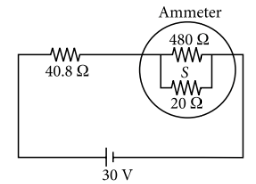Moving coil Galvanometer
Question

# A circuit contains an ammeter, a battery of 30 V and a resistance 40.8 ohm all connected in series. If the ammeter has a coil of resistance 480 ohm and a shunt of 20 ohm, the reading in the ammeter will be

Moderate
Solution

## The circuit shown in the figure.Resistance of the ammeter is${R}_{A}=\frac{\left(480\Omega \right)\left(20\Omega \right)}{\left(480\Omega +20\Omega \right)}=19.2\Omega$$R=40.8\Omega +{R}_{A}=40.8\Omega +19.2\Omega =60\Omega$By Ohm's law, Current in the circuit is Thus the reading in the ammeter will be  0.5  A.

Get Instant Solutions